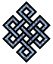fail2ban bad ip database: ip 120.92.15.82

| ip database | live view | stats | report | help | api key:

 ip: 120.92.15.82 hostname: 120.92.15.82 country:[CN] China first reported: 30.10.2018 11:54.00 GMT+0200 last reported: 29.01.2019 05:44.23 GMT+0200 time period: 90d 17h 50m 23s total reports: 11 reported by: 4 host(s) filter(s): ssh (8) ssh (3) tor exit node no badips.com db Lookupport scan of '120.92.15.82':

[-hide]
```# Nmap 6.40 scan initiated Tue Oct 30 11:54:02 2018 as: /usr/bin/nmap -sU -sS -O 120.92.15.82
Nmap scan report for 120.92.15.82
Host is up (0.28s latency).
Not shown: 1000 open|filtered ports, 993 filtered ports
PORT     STATE  SERVICE
20/tcp   closed ftp-data
21/tcp   open   ftp
22/tcp   open   ssh
80/tcp   open   http
443/tcp  closed https
3000/tcp closed ppp
3306/tcp open   mysql
No exact OS matches for host (If you know what OS is running on it, see http://nmap.org/submit/ ).
TCP/IP fingerprint:
OS:SCAN(V=6.40%E=4%D=10/30%OT=21%CT=20%CU=%PV=N%G=Y%TM=5BD83930%P=x86_64-pc
OS:-linux-gnu)SEQ(SP=105%GCD=1%ISR=10A%TI=Z%CI=Z%TS=D)OPS(O1=M5B4ST11NW6%O2
OS:=M5B4ST11NW6%O3=M5B4NNT11NW6%O4=M5B4ST11NW6%O5=M5B4ST11NW6%O6=M5B4ST11)W
OS:IN(W1=3890%W2=3890%W3=3890%W4=3890%W5=3890%W6=3890)ECN(R=Y%DF=Y%TG=40%W=
OS:3908%O=M5B4NNSNW6%CC=Y%Q=)T1(R=Y%DF=Y%TG=40%S=O%A=S+%F=AS%RD=0%Q=)T2(R=N
OS:)T3(R=N)T4(R=Y%DF=Y%TG=40%W=0%S=A%A=Z%F=R%O=%RD=0%Q=)T5(R=Y%DF=Y%TG=40%W
OS:=0%S=Z%A=S+%F=AR%O=%RD=0%Q=)T6(R=Y%DF=Y%TG=40%W=0%S=A%A=Z%F=R%O=%RD=0%Q=
OS:)T7(R=Y%DF=Y%TG=40%W=0%S=Z%A=S+%F=AR%O=%RD=0%Q=)U1(R=N)IE(R=N)

OS detection performed. Please report any incorrect results at http://nmap.org/submit/ .
# Nmap done at Tue Oct 30 11:57:52 2018 -- 1 IP address (1 host up) scanned in 230.58 seconds
```
```Σ = 46 | Δt = 0.003978967666626s
```